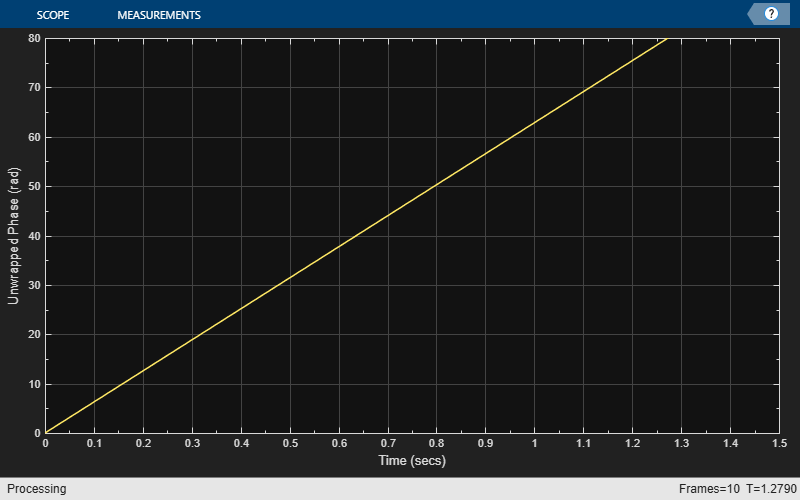dsp.PhaseExtractor

Extract the unwrapped phase of a complex input

Description

The dsp.PhaseExtractor System object™ extracts the unwrapped phase of a real or a complex input.

To extract the unwrapped phase of a signal input:

1. Create the dsp.PhaseExtractor object and set its properties.

2. Call the object with arguments, as if it were a function.

Creation

Description

example

phase = dsp.PhaseExtractor returns a phase extractor System object that extracts the unwrapped phase of an input signal.

example

phase = dsp.PhaseExtractor(Name,Value) returns a phase extractor System object with the specified property name set to the specified value.

Properties

expand all

Unless otherwise indicated, properties are nontunable, which means you cannot change their values after calling the object. Objects lock when you call them, and the release function unlocks them.

If a property is tunable, you can change its value at any time.

Specify if the phase is to be unwrapped only within the frame, as a logical scalar.

When you set this property to:

• false –– The object returns the unwrapped phase while ignoring boundaries between input frames.

• true –– The object treats each frame of input data independently, and resets the initial cumulative unwrapped phase value to zero each time a new input frame is received.

Usage

Description

example

p = phase(input) extracts the unwrapped phase, p, of the input signal. Each column of the input signal is treated as a separate channel. The System object unwraps the phase of each channel of the input signal independently over time.

Input Arguments

expand all

Data input, specified as a vector or a matrix. This object supports variable-size input signals. That is, you can change the input frame size (number of rows) even after calling the algorithm. However, the number of channels (number of columns) must remain constant.

Data Types: single | double
Complex Number Support: Yes

Output Arguments

expand all

Unwrapped phase of the input, returned as a vector or a matrix. The size and data type of the unwrapped phase output match the size and data type of the input signal.

Data Types: single | double

Object Functions

To use an object function, specify the System object as the first input argument. For example, to release system resources of a System object named obj, use this syntax:

release(obj)

expand all

 step Run System object algorithm release Release resources and allow changes to System object property values and input characteristics reset Reset internal states of System object

Examples

collapse all

Note: This example runs only in R2016b or later. If you are using an earlier release, replace each call to the function with the equivalent step syntax. For example, myObject(x) becomes step(myObject,x).

Create a dsp.SineWave System object™. Specify that the object generates an exponential output with a complex exponent.

sine = dsp.SineWave('Frequency',10,...
'ComplexOutput',true,'SamplesPerFrame',128);

Create a dsp.PhaseExtractor System object?. Specify that the object ignores frame boundaries when returning the unwrapped phase.

phase = dsp.PhaseExtractor('TreatFramesIndependently',false);

Extract the unwrapped phase of a sine wave. Plot the phase versus time using a timescope System object.

timeplot = timescope('PlotType','Line','SampleRate',1000,...
'TimeSpanSource','Property','TimeSpan',1.5,'YLimits',[0 80],...
'ShowGrid',true,...
for ii = 1:10
sineOutput = sine();
phaseOutput = phase(sineOutput);
timeplot(phaseOutput)
endNote: If you are using R2016a or an earlier release, replace each call to the object with the equivalent step syntax. For example, obj(x) becomes step(obj,x).

Create a dsp.TransferFunctionEstimator System object™.

tfe = dsp.TransferFunctionEstimator('FrequencyRange','centered');

Create a dsp.PhaseExtractor System object™. Specify that the object must treat each frame of data independently.

phase = dsp.PhaseExtractor('TreatFramesIndependently',true);

Create a dsp.IIRFilter System object™. Compute the transfer function of a third-order IIR filter. Use the butter function to generate coefficients for the filter.

[b,a] = butter(3,.3);
iir = dsp.IIRFilter('Numerator',b,'Denominator',a);

Extract the phase response of the transfer function. Plot using a dsp.ArrayPlot System object™.

sampleRate = 1e3;
phaseplot = dsp.ArrayPlot('PlotType','Line','XOffset',-sampleRate/2,...
'YLimits',[-15 0],...
'XLabel','Frequency (Hz)',...
'Title','System Phase response');
for ii = 1:100
% Generate input
input = 0.05*randn(1000,1);
% Pass through IIR filter
filterOutput = iir(input);
% Estimate transfer function
transferFunction = tfe(input,filterOutput);
% Plot transfer function phase
phaseOutput = phase(transferFunction);
phaseplot(phaseOutput);
endAlgorithms

Consider an input frame of length N:

$\left(\begin{array}{l}{x}_{1}\\ {x}_{2}\\ ⋮\\ {x}_{N}\end{array}\right)$

The object acts on this frame and produces this output:

$\left(\begin{array}{l}{\Phi }_{1}\\ {\Phi }_{2}\\ ⋮\\ {\Phi }_{N}\end{array}\right)$

where:

${\Phi }_{i}={\Phi }_{i-1}+\text{angle}\left({x}_{i-1}^{*}{x}_{i}\right)$

Here, i runs from 1 to N. The angle function returns the phase angle in radians.

If the input signal consists of multiple frames:

• If you set TreatFramesIndependently to true, the object treats each frame independently. Therefore, in each frame, the object calculates the phase using the preceding formula where:

• ${\Phi }_{0}$ is 0.

• ${x}_{0}$ is 1.

• If you set TreatFramesIndependently to false, the object ignores boundaries between frames. Therefore, in each frame, the step method calculates the phase using the preceding formula where:

• ${\Phi }_{0}$ is the last unwrapped phase from the previous frame.

• ${x}_{0}$ is the last sample from the previous frame.

Extended Capabilities